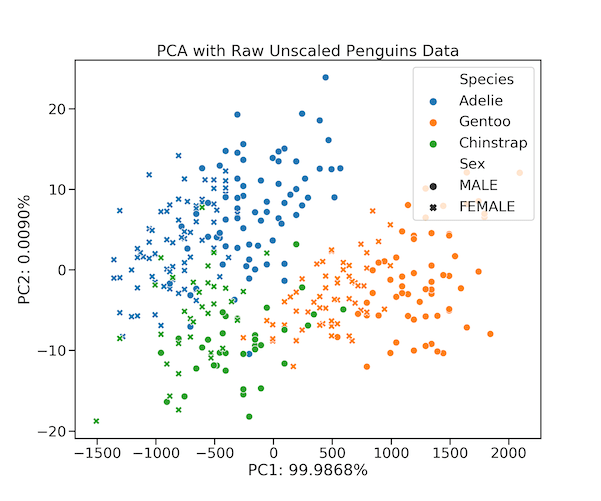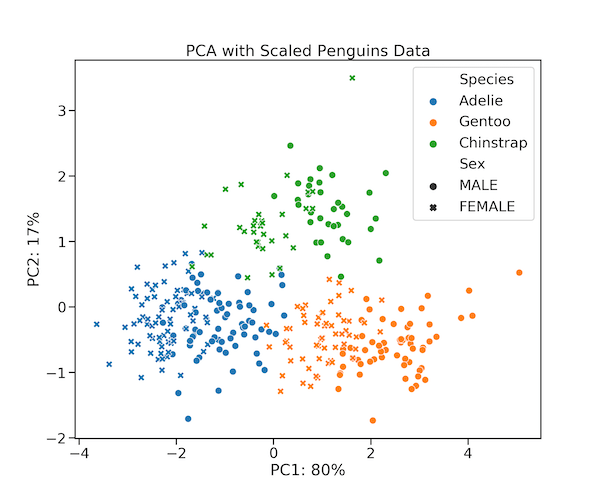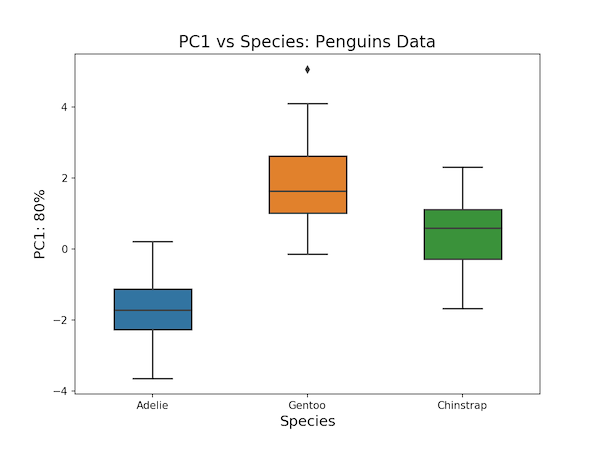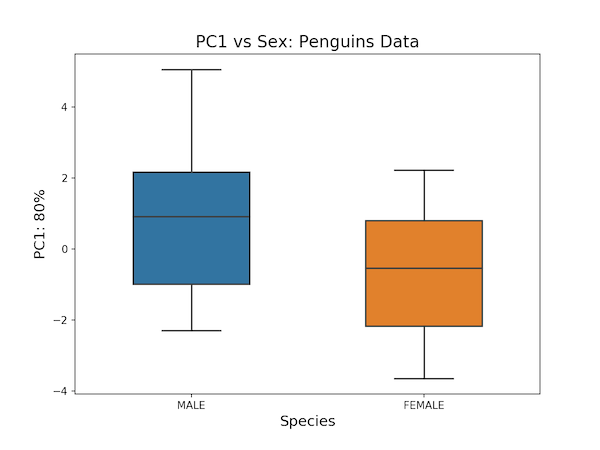# Principal Component Analysis with Penguins Data in Python

381PCA with Penguins Data in Python

Who does not love PCA with Penguins in Python. Sorry, could not resist saying this :). If you are tired of seeing Iris data for introducing all things Machine Learning, Data Science algorithms and Data Visualization examples, you are in for much needed treat in the form of Penguins.

Thanks to Alison Horst, who has made the wonderful dataset readily available for introducing exploratory data analysis, machine learning algorithms and data visualization.

The Penguin Data

were collected and made available by Dr. Kristen Gorman and the Palmer Station, Antarctica LTER, a member of the Long Term Ecological Research Network.

Thank you to Dr. Gorman, Palmer Station LTER and the LTER Network! Special thanks to Marty Downs (Director, LTER Network Office) for help regarding the data license & use.

Let us get started with loading the packages we need. We first load our regular libraries Pandas, numpy, Seaborn, and matplotlib.

```import matplotlib.pyplot as plt
import pandas as pd
import numpy as np
import seaborn as sns
```

We will be using scikit-learn to do Principal Component Analysis with Penguins data. Let us load PCA module from scikit-learn. We will be using scikit-learn’s ability chain together multiple steps of analysis using “pipeline”.

```from sklearn.decomposition import PCA
from sklearn.pipeline import make_pipeline
from sklearn.preprocessing import StandardScaler
```

## Penguins Data: A great dataset to learn Data Visualization, Data Science and Machine Learning

Let us load the raw penguins data from Allison Horst’s github page.

```# path to Penguins data
p2data = "https://raw.githubusercontent.com/allisonhorst/palmerpenguins/master/data-raw/penguins_raw.csv"
```

We can see that the data contains a lot of information about Penguin species.

```penguins_raw = pd.read_csv(p2data)

studyName	Sample Number	Species	Region	Island	Stage	Individual ID
```

We will be mainly using selected columns from the data for our PCA. Let us subset the data

```columns_of_interest = ['Species', "Culmen Length (mm)", "Culmen Length (mm)",
"Flipper Length (mm)", "Body Mass (g)", "Sex"]
penguins_df = penguins_raw.loc[:,columns_of_interest]
```

Note that the names of Penguin species is pretty long. Let us just use simple name describing the Penguin species. And we will also remove any row with missing data.

```# shorten penguin specie name
penguins_df[['Species']]=penguins_df.Species.str.split(" ",expand=True,).loc[:,0]
# replace "." to missing value
penguins_df=penguins_df.replace(".", np.nan)
# drop all rows containing missing value
penguins_df=penguins_df.dropna()
```

Now, we have the data we need for doing PCA with sckit-learn.

```penguins_df.head()
Species	Culmen Length (mm)	Culmen Length (mm)	Flipper Length (mm)	Body Mass (g)	Sex
0	Adelie	39.1	39.1	181.0	3750.0	MALE
1	Adelie	39.5	39.5	186.0	3800.0	FEMALE
2	Adelie	40.3	40.3	195.0	3250.0	FEMALE
4	Adelie	36.7	36.7	193.0	3450.0	FEMALE
5	Adelie	39.3	39.3	190.0	3650.0	MALE
```

Let us subset the data to contain only numeric data for PCA.

```penguins_data=penguins_df.select_dtypes(np.number)
```

And also keep the Penguin species and sex information separate.

```penguins_info=penguins_df.select_dtypes(exclude='float')
Species	Sex
```
```penguins_info.Species.unique()
```
```sex=penguins_info.Sex.tolist()
species=penguins_info.Species.tolist()
```

## PCA with Raw Data

We will first perform PCA with raw data and the do PCA with scaled data to illustrate the importance of scaling the data before doing PCA.

Let us use scikit-learn’s PCA fucntion to do the analysis. We first create a PCA model with 4 components. And then apply fit_transform() function providing the penguins data and perform PCA on the data.

```pca = PCA(n_components=4)
penguins_pca= pca.fit_transform(penguins_data)
```

We have the principal components ready after calling fit_transform() on the PCA model with the data. Let us create a dataframe with principal component

```pc_df = pd.DataFrame(data = penguins_pca ,
columns = ['PC1', 'PC2','PC3', 'PC4'])
PC1	PC2	PC3	PC4
0	-457.339529	12.941050	4.560271	2.259745e-14
1	-407.266928	9.418435	2.184189	-4.475489e-16
2	-957.051463	-6.895631	-5.102509	1.679067e-16
3	-757.136970	0.900180	-6.930255	4.321797e-16
4	-557.188031	4.110899	-1.217727	1.447654e-16
```

And also add the sample level information to the data frame with PCs.

```pc_df['Sex']=sex
pc_df['Species']=species
PC1	PC2	PC3	PC4	Sex	Species
0	-457.339529	12.941050	4.560271	2.259745e-14	MALE	Adelie
1	-407.266928	9.418435	2.184189	-4.475489e-16	FEMALE	Adelie
2	-957.051463	-6.895631	-5.102509	1.679067e-16	FEMALE	Adelie
3	-757.136970	0.900180	-6.930255	4.321797e-16	FEMALE	Adelie
4	-557.188031	4.110899	-1.217727	1.447654e-16	MALE	Adelie
```

Let us first check the variance explained by each Principal Component. We can get the variance explained by each PC from explained_variance_ratio_ method on PCA model. A quick look at the variance show that, the first PC explains all of the variation.

```pca.explained_variance_ratio_
array([9.99867796e-01, 8.99895963e-05, 4.22139074e-05, 2.47920196e-36])
```

Typically, just one PC explaining all the variation is a red flag. You might see such cases, when the features in the data are of very different ranges. Because of that just one variable with huge range could bias the PCA analysis. Clearly, this the case in our example as we have not scaled our data. Note, uou might also see such behaviour when all features are very highly correlated.

Let us just go ahead and making PCA scatter plot with PC1 on x-axis and PC2 on y-axis. We can see that PC1 can separate the species in general.

```import seaborn as sns
plt.figure(figsize=(12,10))
with sns.plotting_context("notebook",font_scale=1.25):
sns.scatterplot(x="PC1", y="PC2",
data=pc_df,
hue="Species",
style="Sex",
s=100)
```PCA Plot with Penguin Unscaled Data

## PCA with Scaled Data

Now that we have seen an example of PCA on raw data without scaling, Let us do PCA on data set that is scaled. In Scikit-learn we can use StandardScalar() function to scale the data into data with mean zero and variance one. We will do PCA on the scaled data.

We can use Scikit-learn’s make_pipeline() to create a pipeline with these two steps.

```random_state = 0
pca_scaled = make_pipeline(StandardScaler(),
PCA(n_components=4, random_state=random_state))
```

Let us use fit_transform() on the pipeline for PCA with scaled data.

```penguins_pc_scaled=pca_scaled.fit_transform(penguins_data)

```

We can take a quick peek at the scaled data that is used for PCA

```pca_scaled.named_steps['standardscaler'].fit_transform(penguins_data)
array([[-0.89604189, -0.89604189, -1.42675157, -0.56847478],
[-0.82278787, -0.82278787, -1.06947358, -0.50628618],
[-0.67627982, -0.67627982, -0.42637319, -1.1903608 ],
...,
[ 1.02687621,  1.02687621, -0.56928439, -0.53738048],
[ 1.24663828,  1.24663828,  0.64546078, -0.13315457],
[ 1.13675725,  1.13675725, -0.2120064 , -0.53738048]])
```

Also we can also check what is in the PCA step of the pipeline.

```pca_scaled.named_steps['pca']
PCA(copy=True, iterated_power='auto', n_components=4, random_state=0,
svd_solver='auto', tol=0.0, whiten=False)
```

Let us get the proportion of variation explained by each principal component.

```pca_scaled.named_steps['pca'].explained_variance_ratio_*100
array([7.95338843e+01, 1.73923807e+01, 3.07373502e+00, 2.79398725e-35])
```

We can see that the first PC explains 80 percent of the variation in the data and the second PC explains about 18% of the variation.

Let us create data frame with PCs from scaled data and also add the Penguin infomation for each sample.

```pc_scaled_df = pd.DataFrame(data = penguins_pc_scaled ,
columns = ['PC1', 'PC2','PC3', 'PC4'])
pc_scaled_df['Species'] = species
pc_scaled_df['Sex'] = sex

PC1	PC2	PC3	PC4	Species	Sex
0	-1.899358	0.105560	0.588102	8.403066e-18	Adelie	MALE
1	-1.616865	-0.022060	0.373257	4.885022e-19	Adelie	FEMALE
2	-1.472415	0.213019	-0.547795	1.644519e-18	Adelie	FEMALE
3	-2.101064	-0.501786	-0.334550	-4.239310e-19	Adelie	FEMALE
4	-1.601048	-0.082743	0.033770	8.429747e-19	Adelie	MALE
```

Now we are ready to make visualization using PCA result. Let us first make scatter plot between PC1 and PC2, the two PCs that captures most the variations in the data. We also color the data points by species and change shape of data points by Sex.

```plt.figure(figsize=(12,10))
with sns.plotting_context("talk",font_scale=1.25):
sns.scatterplot(x="PC1", y="PC2",
data=pc_scaled_df,
hue="Species",
style="Sex",
s=100)
plt.xlabel("PC1: "+f'{var_explained:.0f}'+"%")
plt.ylabel("PC2: "+f'{var_explained:.0f}'+"%")
plt.title("PCA with Scaled Penguins Data")
plt.savefig("PCA_plot_PC1_vs_PC2_Penguins_scaled_data.png",
format='png',dpi=150)
```

We can see that the PCA plot with scaled data nicely captures the structure in the data. The data points corresponding to each Penguin species is clearly clustered and well separated compared to the PCA plot with unscaled data. We can also see the effect of Sex more clearly now, as females weigh less compared to males on an average..PCA Plot with Penguin Scaled Data

With little of squinting the scatter plot between PC1 and PC2 we can see how PC1 and PC2 independently has captured the variation between the species and sex. We can do a better job of understanding this by visualizing the PCs together with variables of interest.

At first, let us make a boxplot with species on x-axis and PC1 on y-axis. This would tell how much of the variation captured by PC1 is due to Species level differences in our data.

```sns.plotting_context("talk",font_scale=1.25)
plt.figure(figsize=(8,6))
sns.boxplot(x="Species",y="PC1",
width=0.5,
data=pc_scaled_df)
plt.xlabel("Species", size=14)
plt.ylabel("PC1: "+f'{var_explained:.0f}'+"%", size=14)
plt.title("PC1 vs Species: Penguins Data", size=16)
plt.savefig("PCA_plot_PC1_vs_Species_Penguins_scaled_data.png",
format='png',dpi=150)
```

We can clearly see how PC1 has captured the variation at Species level. FIrst principal component is telling how Adlie Penguins are different from the other two species.PCA Plot: PC1 vs Species Scaled Data

Let us make boxplot between PC1 and Sex. Remember, PC2 captures about 18% pf the variation in the data.

```plt.figure(figsize=(8,6))
sns.boxplot(x="Species",y="PC2",
width=0.5,
data=pc_scaled_df)
plt.xlabel("Species", size=14)
plt.ylabel("PC2: "+f'{var_explained:.0f}'+"%", size=14)
plt.title("PC2 vs Species: Penguins Data", size=16)
plt.savefig("PCA_plot_PC2_vs_Species_Penguins_scaled_data.png",
format='png',dpi=150)
```

We can see that PC2 captures the difference between how Chinstrap species is different from the other two species.PCA Plot: PC2 vs Species Scaled Data

As principal components are linear combination of the original variables, PCs can also be correlated with other variables in the data. For example, if we make a boxplot between PC1 and Sex, we can see that Sex is correlated with PC1, showing that PC1 also captures the variation due to Sex.

We can get this information in our PCA plot as well, by squintingPCA Plot: PC1 vs Species Scaled Data

If we make a boxplot bweet Sex and PC2, we can see that they is no association suggesting that PC2 does not explain Sex.

In summary, in this post we used a fantastic new data set on Penguin species. And showed how to do PCA with Python’s Scikit-learn tootlkit. More importantly, we learned how scaling the data matters by performing PCA without scaling the data and after scaling the data. And we also learned of ways to interpret the PCs obtained from the PCA analysis.

The post Principal Component Analysis with Penguins Data in Python appeared first on Python and R Tips.